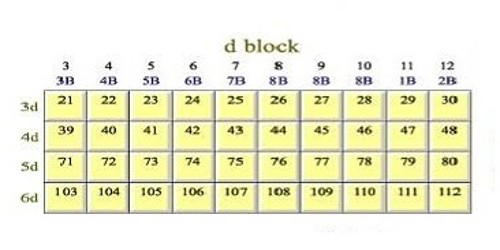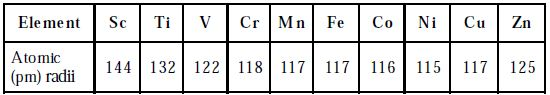# What are Atomic and Ionic Radii of D-Block Elements

Atomic and Ionic Radii of D-Block Elements:

The atomic and ionic radii of transition elements are smaller than those of ‘p’ block elements and larger than those of s-block elements. The atomic and ionic radii of first transition elements are given in the Table. All the d-block elements have the same number of electrons in the outermost shell. Hence, they show similar chemical properties.

Table: Atomic radii of elements of first transition seriesAtomic and ionic radii of elements of all three-transition series. These series are characterized by the completely filled 3d, 4d, and 5d subshells and are named as 3d-(first series) Sc – Zn, 4d series (second series) Y-Cd and the 5d series (third series) La- Hg respectively.

• Decreases rapidly, from column 3 to 6
• Remains steady, from column 7 to 10 and
• Start increasing from column 11 to 12.

Atomic radii: a great degree of variation is seen in the atomic radii across each transition series. The atomic radii of the d-block elements within a given series decrease with an increase in the atomic number. This is due to the increase in the nuclear charge that attracts the electron cloud inwards resulting in a decrease in size.

Ionic radii: The ionic radius is similar to the pattern of atomic radii. The pattern of the ionic radius is similar to the atomic radii pattern. Thus, for ions of a given charge, the radius decreases gradually with increase in atomic number. Hence, for ions of a given charge, the radius decreases gradually with growth in the atomic number.

The atomic radii of the first transition series decrease from Sc to Cr and remain almost constant till Cu and then increases towards the end. This can be explained based on two effects namely screening and the nuclear charge effect. These two effects oppose each other resulting in an increase in nuclear charge. Hence very slight variation in the atomic radii from Cr to Cu is observed. For example, in the first transition series, atomic radii, the decrease is more from Sc to Cr (group 3 to 6 ), is almost the same for Mn, Fe, Co, Ni (group 7,8 9 &10) and increase in Cu and Zn. The larger decrease in atomic radii, in column 3 to 6 elements is due to the increase in effective nuclear charge but poor shielding because of the smaller number of d-electrons.

It has been observed that Zirconium and Hafnium have almost equal atomic radii. In elements of column 7 to 10 increasing effective nuclear charges are balanced by the repulsion between the shared d electrons so that radii remain the same. This is because of the lanthanide contraction. This higher nuclear charge leads to the larger shrinkage of radii known as Lanthanide contraction.